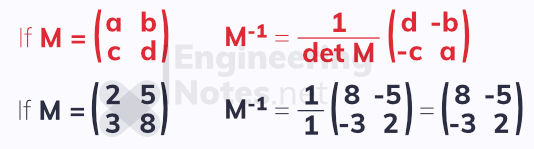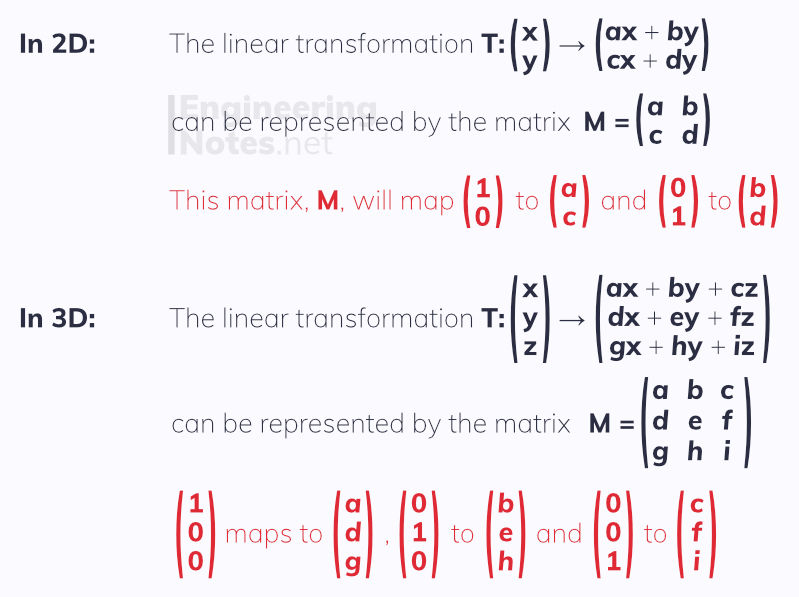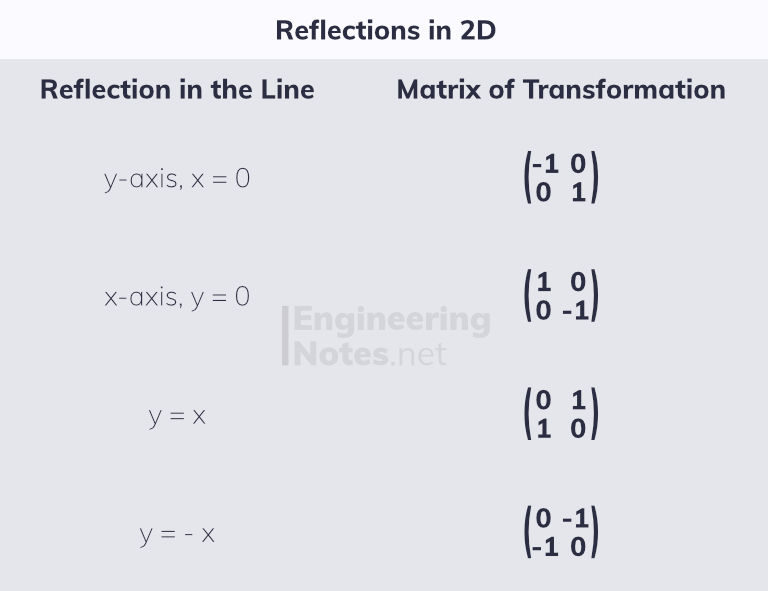top of page

#### Notes by Category University Engineering

Rate these notesNot a fanNot so goodGoodVery goodBrillRate these notes

# Matrices & Linear Transformations

A matrix is a system of elements within a pair of brackets. The size of the matrix is given as the number of rows and columns in it.• A square matrix is one where there is an equal number of rows and columns

• A zero matrix is one in which all the elements are zero

• An identity matrix is a square matrix where the all the values on the diagonal from top left to bottom right are 1, and all other values are zero. This is noted as capital i, I with a subscript number afterwards to show its size.

## Working with Matrices

To add or subtract matrices, you add or subtract the corresponding elements in each matrix.

You can only add or subtract matrices of the same size### Multiplying by a Scalar

To multiple a vector by a scalar, simply multiple each element in the vector by the scalar. This can be factorised out, too.### Multiplying One Vector by Another

Matrix multiplication is only possible if the number of columns in the first matrix equals the number of rows in the second matrix. The result of the multiplication is known as the product matrix, and will have the same number of rows as the first matrix and the same number of columns as the second matrix.

Order of matrix multiplication matters

For two matrices, A and B:

• In general, AB ≠ BA

• If AB exists, it does not mean BA exists

To find the product of two matrices, multiply the elements in each row of the first matrix by the elements in each column of the second matrix## Determinants

The determinant of a square matrix is a scalar value that represents the matrix. It is only possible for square matrices.

• The determinant of a matrix, M, is written as det M, or as |M|

• A singular matrix has a determinant of zero.

• A non-singular matrix has a determinant that is not zero.

To find the determinant of a 2x2 matrix:To find the determinant of a 3x3 matrix:## Inverse Matrices

The inverse of a matrix M is the matrix M¯¹

M M¯¹ = M¯¹ M = 1

### Finding the Inverse of a 2x2 Matrix1. Find the determinant

2. Write out the matrix where a and d are swapped, and both b and c are multiplied by -1

3. Multiply by one over the determinant

If A and B are non-singular matrices, (AB)¯¹ = B¯¹A¯¹

### Finding the Inverse of a 3x3 Matrix1. Find the determinant

2. Replace each element with it matrix of minors & find the determinants

3. Form the matrix of co-factors (switch every other sign)

4. Find the transpose of the matrix of co-factors by switching rows and columns

5. Multiply this by one over the determinantSince this is such a complicated thing to do, unless specifically needed, use the calculator function.

On the CASIO ClassWiz fx-991EX:

1. Click MODE

2. Click 4: Matrix

3. Click 1: Define Matrix A

4. Click 3 twice to set size of matrix

5. Fill in Matrix

6. Click AC

7. Click OPTN

8. CLICK 3: Matrix A

9. Click x¯¹

10. Click =

## Matrix Systems & Geometry

It is possible to use matrices and their inverses to easily solve simultaneous equations.This example is consistent, meaning there is at least one set of values that satisfies all three equations simultaneously. The determinant is not zero, making the matrix non-singular.

This is especially useful for 3D geometry, and the equations of planes:## Linear Transformations

A linear transformations are transformations with specific properties:

• they are made up of linear x, y and z terms only

• they have no non-variable terms

• they map the origin onto itself

• they can be represented by matricesPoints and lines that do not change in a transformation are called invariant points/lines
• The origin is always an invariant point.

• Every point on an invariant line is an invariant point.

### Reflections

The line of reflection is always an invariant line.

In two-dimensions, there are standard reflections for the coordinate axes and y=±x.In three-dimensions, reflections ca happen in planes:For a linear transformation with matrix M, if the determinant is negative, the shape has been reflected

### Rotations

For rotations about the origin, the only invariant point is the origin.In 3D, rotations can be about an entire axis:### Enlargements & Stretches• If a = b, the transformation is an enlargement of scale factor a.

• For a stretch that is only in the x-direction, the y-axis is an invariant line.

• For a stretch that is only in the y-direction, the x-axis is an invariant line.

• For stretches in both directions, there are no invariant lines and the only invariant point is the origin.

For a linear transformation with matrix M, the determinant is the area scale factor.

### Successive & Inverse Transformations

It is possible to apply multiple transformations, one after the other. In this instance, order of matrix multiplication is particularly important.

For two linear transformations, represented by the matrices P and Q respectivley:

PQ represents the transformation Q followed by P

A linear transformation with matrix A can be undone with the transformation represented by the inverse matrix, A¯¹.

See All
bottom of page